Electrics Index

Electrical Units

Introduction

 Unit (Symbol) Description Unit Abrev. Ampere (I) The constant current which when flowing through two infinitely long parallel wires 1 m apart would produce in vacua a force of 2 x 10 -7 N/m on each conductor A Coulomb (Q) The quantity of electricity passing a given point when a current of 1 ampere flows for 1 second C Volt (E,V) The electromotive force or potential difference across a resistor which produces heat at the rate of 1 newton.m (joule)/second, =1 watt, when a constant current of 1 Ampere is flowing. V Ohm (R) Is the electrical resistance of a conductor in which a constant current of 1 ampere flows under a constant voltage of 1 volt. ( R = V /I ) Ω (ρ) Resistivity . The resistance of a conductor ( R ) is proportional to the length ( l ) / Area( A ) and the proportionality constant is the resistivity ρ. i.e R = ρ. l / A. Ω.m Siemen (G) The unit of conductance G = The Siemen .    The Sieman is the conductance between two points on a conductor when a constant potential difference of 1 volt produces a currant of 1 amperere .    (G = I / V ) S (σ) Conductivity .    The conductivity of a conductor ( G ) is proportional to the area / length and the proportionality constant is the conductivity σ. i.e G = σ. l / A.    Conductivity is the inverse of resistivity S.m Henry (L) The inductance in which 1 volt is produced by a current changing at the rate of one  ampere  per  second H Farad (C) The capacitance in which 1 coulomb is stored for a potential difference of 1 volt F Resistivity (ρ) The unit of static resistivity.     The resistance of a conductor (Ω) is proportional to the length ( l ) / Area( A ) and the proportionality constant is the resistivity ρ. i.e R = ρ. l / A.    Resistivity is the inverse of conductance σ S Joule (W) The unit of work energy, and quantity of heat.    The work done when the point of application of a force of 1 newton is displaced through a distance of 1 m. in the direction of the force J Watt (P) The unit of power . One watt = The work of one joule being completed per second . (P = W/t) W Weber (Φ) The unit of magnetic flux. The magnetic flux linking a circuit of one turn produces in it an electromotive force of 1 volt as it is reduced to zero at a uniform rate in 1 second Wb Tesla (B) The unit of magnetic flux density. The tesla is equal to 1 weber per square metre of circuit area T

Definitions in a little more detail

Time...(t) (Seconds).... Length...(l) (metres).... Mass..(m) (kilograms).... Velocity ..(v) (m/s)....

Force...(F) .. A force of one newton is required to cause a mass of 1 kilgram to accelerate at a rate of 1 metre per second per second.

Energy....(W).. One joule is the energy required (the work done) in moving a object 1 meter against a resisting force of 1 newton.   Potential energy (mechanical) is the capacity of a body to do work because of its position in a force field.  Kinetic energy is the energy possessed by a body due to its motion (KE= m.v2 /2). i.e a mass of 1 kg moving with a velocity of 1 m/s has a kinetic energy of 1/2 joule.
Using electrical units a charge of 1 coulomb gains an energy of 1 joule in moving through a potential difference of 1 Volt.

Power..(P).. One watt is the power required to do the work of 1 joule in one second.   Instantaneous power P = dW/dt .
In electrical units a flow of charge of couloumb/sec ( = a current of 1 Ampere) flowing against a potential difference of 1 volt requires a power of 1 watt.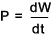Charge..(Q)..(SI derived unit ) A charge is the electrostatic force resulting from presence of electrons.  This is a quantized variable because the smallest charge is that resulting from 1 electron (1,602.10-19 Coulomb).  The Coulomb is the charge on 6,24.1018 electrons or as a charge experiencing a force of 1 Newton in a electric field of 1 Volt per metre , or as the charge transferred in 1 second at a current of 1 Ampere.   (Q = I.t )

Current ...(i)..(SI base unit )The current through an area A is defined by the electric charge passing through A per unit of time.  An Ampere is the charge flow of 1 coulomb per second.  In general the charges may be positive and negative each moving moving through the area in opposite directions.  The current is the net rate of flow of positive charges, a scalar quantity.  For example if positive charges are moving to the right and negative charges are moving to the left then the net effect is the sum of the rate of positive charges and the rate of negative charge, moving to the right..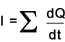Potential Difference (PD)..(V)..(SI derived unit ) .The energy transfer of a flow of electric charge is determined by the potential difference of voltage through which the charge moves.  A charge of 1 coulomb acquires or delivers an energy of 1 joule in moving through a potential difference of 1 volt.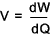Electro Motive Force (EMF)..(V)..(SI derived unit ) .The force which produce an electric current.  The potential difference in Volts developed by a generator, battery etc. An e.m.f can be produced by

Chemical action in batteries or cells
Mechanical energy using magnetic induction / piezo-electric crystals .
Thermal energy using thermocouples.
Solar energy using solar cells.

Electric Field Strength ..(Ε)..The field is a convenient concept in calculating electric and magnetic forces.  Around a charge we imagining a region of influence called an " electic field".  The electric field Ε is a vector defined by the magnitude and direction of the force (F) on a unit positive charge in the field   In vector notation the defining equation is F = Q .Ε .   The units of electric field strength are newtons per coulomb .

[force/charge = force.distance /charge.distance = energy /charge.distance = voltage /distance ]

The field strength in newtons per coulomb is equal and opposite to the voltage gradient.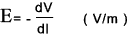Magnetic Flux Density..(B)...(SI derived unit ).  Around a moving charge or an electric current there is a region of influence called a "magnetic field".  The magnetic field associated with a bar magnet results from spinning electrons in the atoms of iron.  The intensity of the magnetic field is measured by the magnetic flux density B is measured in teslas(T).  It is defined by the magnitude and direction of the force exerted on a charge (Q) moving in a field with a velocity (v) .  In vector notation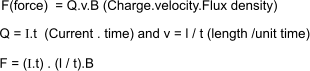That is a force of 1 newton is experienced by a charge of 1 coulomb moving with a velocity of 1 m/s normal to a magnetic flux density of 1 tesla .   Also a force of 1 newton is experienced by a conductor, normal to the magnetic field, carrying a current of 1 ampere per metre length of conductor

Magnetic Flux ..Φ.. Historically ,magnetic fields have been described in terms of lines of force of flux.  The magnetic flux Φ measured in Webers (Wb) are obtained by integrating magnetic flux density over an area.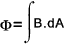Useful Electrical Links SI units � Electricity and magnetism ... Names of units Glossary of electrical terms... includes unit descriptions Definitions of the SI Units and Other Units of Measurement... Site provides quality information SI Units An excellent source of information

Electrics Index